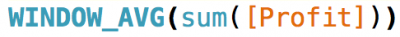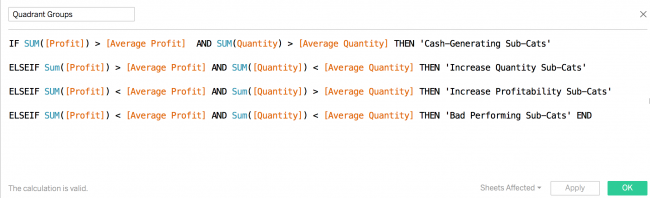Alteryx

# Improving Your Scatterplot - Part II

In this blog-post, I am going to show how you can color each of your quadrants according to the average of the two measures in your view.

This is useful as your can categorize your customers according these two measures. You can then go on and apply different strategies when targeting each group.

I have made a packaged workbook with the example here: Improving Your Scatterplot - Part II

### Initial View

First you need to construct the view that you are interested in. In this case, I plot profit and quantity by each customer. Additionally, I add reference lines, showing the average of each measure. These are the ones that are supposed to split my customers into the quadrant-groups. This results in the following view:The next step is to make use of the window_average-function, which can be used to calculate the average of a given measure displayed in the view. I create two new calculated fields returning the window_average of both Profit and Quantity:This now enables me to use these in a third calculated field, which defines each group related to the quadrants. I go ahead and create the field called Quadrant Groups:The calculation looks more extensive than what is actually the case - but go ahead and try it out yourself. Now, having created the Quadrant Groups field, we can go on and apply it to the view:

As the new field contains window_averages - thus table calculations - we need to define it to Compute Using: Customer Name, in order to get the coloring right.
Last step would be to brush up the layout a bot by applying borders and changing the opacity:

Back to blog